# JP4823979B2 - Image processing apparatus and method, and program - Google Patents

## Info

Publication number
JP4823979B2
JP4823979B2 JP2007190529A JP2007190529A JP4823979B2 JP 4823979 B2 JP4823979 B2 JP 4823979B2 JP 2007190529 A JP2007190529 A JP 2007190529A JP 2007190529 A JP2007190529 A JP 2007190529A JP 4823979 B2 JP4823979 B2 JP 4823979B2
Authority
JP
Japan
Prior art keywords
correction
image
area
value
calculating
Prior art date
Legal status (The legal status is an assumption and is not a legal conclusion. Google has not performed a legal analysis and makes no representation as to the accuracy of the status listed.)
Active
Application number
JP2007190529A
Other languages
Japanese (ja)
Other versions
JP2009027583A (en
Inventor

Original Assignee

Priority date (The priority date is an assumption and is not a legal conclusion. Google has not performed a legal analysis and makes no representation as to the accuracy of the date listed.)
Filing date
Publication date
Application filed by 富士フイルム株式会社 filed Critical 富士フイルム株式会社
Priority to JP2007190529A priority Critical patent/JP4823979B2/en
Publication of JP2009027583A publication Critical patent/JP2009027583A/en
Application granted granted Critical
Publication of JP4823979B2 publication Critical patent/JP4823979B2/en
Active legal-status Critical Current
Anticipated expiration legal-status Critical

• 238000004364 calculation methods Methods 0.000 claims description 73
• 238000000034 methods Methods 0.000 claims description 14
• 230000001186 cumulative Effects 0.000 claims description 9
• 238000003672 processing method Methods 0.000 claims description 6
• 230000001815 facial Effects 0.000 claims 1
• 238000003384 imaging method Methods 0.000 description 18
• 238000010586 diagrams Methods 0.000 description 12
• 102100004256 F-box-like/WD repeat-containing protein TBL1Y Human genes 0.000 description 8
• 101710045666 TBL1 Proteins 0.000 description 8
• 101710076481 TBL1X Proteins 0.000 description 8
• 101710076475 TBL1Y Proteins 0.000 description 8
• 101710003985 TCP18 Proteins 0.000 description 8
• 238000007781 pre-processing Methods 0.000 description 7
• 230000001629 suppression Effects 0.000 description 6
• 239000010936 titanium Substances 0.000 description 6
• 230000000875 corresponding Effects 0.000 description 5
• 101710076239 TBL11 Proteins 0.000 description 4
• 101710076240 TBL12 Proteins 0.000 description 4
• 101710045649 TBL4 Proteins 0.000 description 4
• 239000011651 chromium Substances 0.000 description 3
• 230000037242 Cmax Effects 0.000 description 2
• 230000012447 hatching Effects 0.000 description 2
• 239000010955 niobium Substances 0.000 description 2
• 238000005457 optimization Methods 0.000 description 2
• 102100012032 C-C motif chemokine 20 Human genes 0.000 description 1
• 102100005660 Krueppel-like factor 6 Human genes 0.000 description 1
• 238000009825 accumulation Methods 0.000 description 1
• 239000003086 colorants Substances 0.000 description 1
• 238000007906 compression Methods 0.000 description 1
• 230000002349 favourable Effects 0.000 description 1
• 238000010801 machine learning Methods 0.000 description 1
• 229910044991 metal oxides Inorganic materials 0.000 description 1
• 238000010606 normalization Methods 0.000 description 1
• 238000003825 pressing Methods 0.000 description 1
• 239000004065 semiconductors Substances 0.000 description 1

## Images

•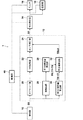•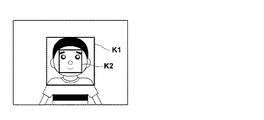••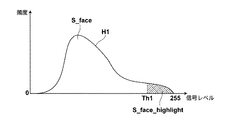•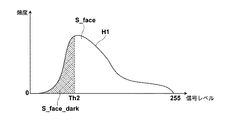•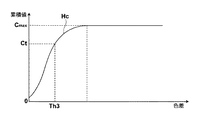•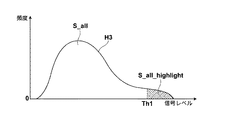••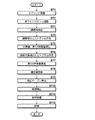•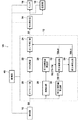•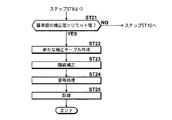•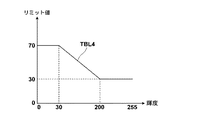•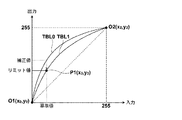••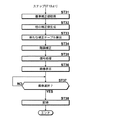••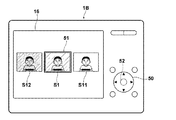## Description

The present invention relates to an image processing apparatus and method for performing image processing on an image acquired by an imaging apparatus such as a digital camera, and a program for causing a computer to execute the image processing method.

The image quality of an image is improved by performing image processing such as gradation processing on an image acquired by an imaging device such as a digital camera or an image reading device such as a scanner.

For example, the reliability and backlight intensity of the image is determined based on the brightness information of the person included in the image, and the reliability and character level of the person scene are determined, and the image level is determined based on the information. A technique for performing tone correction has been proposed (see Patent Document 1). Further, a face area is detected from the image, a correction table for correcting the image is calculated based on the brightness value of the face area and the luminance distribution information of the entire area of the image, and the correction table is used to calculate the image. There has been proposed a method for correcting the color tone (see Patent Document 2). According to the technique described in Patent Literature 2, it is possible to make the gradation of the face preferable in an image including a person, and it is also possible to set the background of the face to a preferable gradation.
JP-A-8-62741 JP 2007-124604 A

However, the face of a person included in the image may include not only a uniform brightness but also an area that becomes a highlight or shadow, and the contrast may change within the face area. Also, the entire area of the image may include an area that becomes a highlight or a shadow, and the contrast of the entire image may also change. For this reason, using only the brightness of the entire area of the face or image as in the methods described in Patent Documents 1 and 2, for example, in the case of a face with contrast, the face becomes too bright due to overcorrection. May end up.

The present invention has been made in view of the above circumstances, and an object thereof is to allow an image to be corrected appropriately in consideration of various situations of a specific region such as a face included in an image and the entire region of the image. .

An image processing apparatus according to the present invention includes a first feature amount calculating unit that calculates a representative value of a specific region included in an input image and a plurality of first feature amounts respectively representing a plurality of features of the specific region. When,
A second feature amount calculating means for calculating at least one second feature amount representing the feature amount of the entire area of the image;
And a correction value calculating means for calculating a correction value for correcting the image based on the representative value, the plurality of first feature amounts, and the second feature amount. is there.

In the image processing apparatus according to the present invention, the correction value may be a target value of the representative value of the specific area.

The image processing apparatus according to the present invention may further include detection means for detecting the specific area from the image.

In the image processing apparatus according to the present invention, the first feature amount calculating means calculates the luminance and contrast of the specific region and the ratio of the frequency of the specific luminance in the specific region as the first feature amount. It may be a means.

In the image processing apparatus according to the present invention, the second feature amount calculating means may be configured to calculate at least one of the luminance, contrast, and color difference of the entire area and the frequency ratio of the specific luminance in the entire area. It is good also as a means to calculate as a feature-value.

In the image processing apparatus according to the present invention, the first feature amount calculation unit may be a unit that calculates the representative value from either the luminance of the specific region or each color component.

In the image processing apparatus according to the present invention, the correction value calculation unit calculates the correction value by applying the first feature amount and the second feature amount to an arithmetic expression determined by a predetermined parameter. It is good also as a means to do.

In this case, the predetermined parameter may be a parameter set based on an evaluation experiment using a plurality of sample images.

In the image processing apparatus according to the present invention, the correction value calculation unit may be a unit that calculates a correction table based on the correction value.

In this case, it is determined whether or not the correction of the image by the correction table is overcorrected, and when the determination is affirmative, a correction value for calculating a new correction table in which the correction amount is suppressed compared to the correction table It may be further provided with a correcting means.

Also, in this case, the correction value obtained by correcting the correction value correction means to determine whether or not the overcorrection is performed using the correction table to correct the reference value in the image is a predetermined threshold. Means for determining whether or not the correction is larger than the value may be used.

The image processing apparatus according to the present invention may further include a correcting unit that corrects the image data based on the correction table.

The image processing apparatus according to the present invention may further include a correction table calculating unit that calculates at least one other correction table having a degree of correction different from that of the correction table based on the correction table.

In this case, correction means for correcting the image data based on the correction table and the other correction table to obtain a plurality of processed image data;
Display means for displaying a plurality of processed images represented by the plurality of processed image data;
The image processing apparatus may further include selection means for receiving selection of a desired processed image from the plurality of processed images.

In the image processing apparatus according to the present invention, the first feature amount calculating unit may be configured to calculate the plurality of first feature amounts based on pixel value distribution information of the specific region in the image. Also good.

In the image processing apparatus according to the present invention, the second feature amount calculating unit may be a unit that calculates the second feature amount based on pixel value distribution information of the entire region in the image. .

The image processing method according to the present invention calculates a representative value of a specific area included in an input image and a plurality of first feature amounts respectively representing a plurality of features for the specific area,
Calculating at least one second feature amount representing the feature amount of the entire region of the image;
A correction value for correcting the image is calculated based on the plurality of first feature amounts and the second feature amount.

The image processing method according to the present invention may be provided as a program for causing a computer to execute the image processing method.

According to the present invention, the representative value of the specific region included in the input image and the plurality of first feature amounts respectively representing the plurality of features of the specific region are calculated, and the feature amount of the entire region of the image is represented. At least one second feature amount is calculated. Then, a correction value for correcting the image is calculated based on the representative value, the plurality of first feature amounts, and the second feature amount. As described above, in the present invention, the correction value is calculated based on a plurality of feature amounts in the specific area, so that the correction value is calculated based on only one feature amount such as luminance. Thus, the specific area can be corrected more appropriately. Further, since the feature amount of the entire area of the image is taken into consideration, not only the specific area but also the entire image can be corrected appropriately.

Further, by setting the correction value as the target value of the representative value of the specific area, when the image is corrected with the correction value, the representative value of the specific area can be set as the target value.

Further, by detecting the specific area from the image, it is not necessary for the user of the image processing apparatus of the present invention to specify the specific area on the image, so that the burden on the user can be reduced.

In addition, by calculating the ratio of the luminance and contrast of the specific area and the frequency of the specific luminance in the specific area as the first feature amount, the luminance of the specific area, the contrast and the ratio of the frequency of the specific luminance are considered, The brightness and contrast of the specific area can be made more preferable. In particular, when the specific area is a face, the brightness and contrast of the face can be made more preferable.

Further, by calculating at least one of the luminance, contrast, color difference of the entire area of the image and the ratio of the frequency of the specific brightness in the entire area as the second feature amount, the brightness, contrast, color difference of the entire image, and the specific frequency are calculated. Considering the ratio of luminance frequency, the brightness, contrast or color of the entire area of the image can be made more preferable.

Further, the correction value can be easily calculated by applying the first feature amount and the second feature amount to an arithmetic expression determined by a predetermined parameter.

In this case, a robust correction value can be calculated by setting the predetermined parameter to a parameter set based on an evaluation experiment using a plurality of sample images.

Further, the image can be easily corrected by using the correction table calculated based on the correction value.

In addition, when the correction of the image by the correction table is overcorrection, it is possible to prevent the image from being overcorrected by calculating a new correction table in which the correction amount is suppressed from that of the correction table. .

By calculating at least one other correction table having a degree of correction different from that of the correction table, processed images having various degrees of correction can be obtained.

In this case, processed images having various degrees of correction are displayed, and a processed image having a desired image quality can be obtained by receiving selection of a desired processed image. Further, since it is only necessary to record the selected processed image, it is possible to prevent the consumption of the capacity of the recording medium for recording the image.

Hereinafter, embodiments of the present invention will be described with reference to the drawings. FIG. 1 is a schematic block diagram showing the configuration of a digital camera to which the image processing apparatus according to the first embodiment of the present invention is applied. In the digital camera 1 shown in FIG. 1, illustration and description of portions not directly related to the present invention are omitted.

As shown in FIG. 1, the digital camera 1 includes an imaging unit 10, a preprocessing unit 12, a signal processing unit 14, a monitor 16, and a recording unit 18.

The imaging unit 10 includes an imaging element in which a plurality of light receiving elements (not shown) that convert incident light into an electrical signal are two-dimensionally arranged, and has a function of reading a signal converted by the imaging element. Examples of the imaging element include a CCD (Charge Coupled Device) type or a C-MOS (Complimentary-Metal Oxide Semiconductor) type, and may be other signal readout type.

In addition, the imaging unit 10 acquires an analog imaging signal obtained corresponding to each light receiving element of the imaging element in accordance with a drive signal supplied from a driving unit (not shown), and removes noise from the analog imaging signal A function of adjusting the gain of the analog imaging signal (hereinafter referred to as analog processing), converting the analog processed analog imaging signal into a digital signal, and outputting the digital image data S0 to the preprocessing unit 12 Also have. Here, in the present embodiment, it is assumed that the image data S0 has an 8-bit gradation. In the following description, S0 is used as a reference symbol for the image represented by the image data S0.

The preprocessing unit 12 includes an offset unit 20, a white balance (WB) adjustment unit 22, a gamma correction unit 24, a face detection unit 26, a first feature amount calculation unit 28, a second feature amount calculation unit 30, and a correction value. A calculation unit 32 is provided.

The offset unit 20 has a function of adjusting the pixel values corresponding to each pixel of the offset adjustment, that is, the digital image data S0 to a predetermined level.

The WB adjustment unit 22 has a function of adjusting white balance for the image data S0 input from the offset unit 20.

The gamma correction unit 24 has a function of correcting the gradation of the image data S0 input from the WB adjustment unit 22 using a correction table TBL0 supplied from a correction value calculation unit 32 described later. Note that the gamma correction unit 24 outputs the image data S <b> 1 whose tone has been corrected to the signal processing unit 14. The image data S1 is RAW data having a color component corresponding to the light receiving element of the imaging element in the imaging unit 10 for each pixel. In the following description, S1 is used as a reference symbol for the image represented by the image data S1.

The face detection unit 26 has a function of detecting all face regions included in the image S0. Specifically, the face detection unit 26 uses a template matching method, a method using a face discriminator obtained by machine learning learning using a large number of sample images of the face, and the like to enclose a predetermined face on the image S0. A range area is detected as a face area.

The first feature amount calculation unit 28 sets the representative value R0 of the face area included in the image S0, the brightness of the face area, the degree of contrast, the ratio of highlight of the face area, and the ratio of dark part of the face area as the feature amounts T1 to T1, respectively. Calculated as T4. The feature amounts T1 to T4 are the first feature amounts. Hereinafter, the calculation of the feature amounts T1 to T4 will be specifically described.

Here, the face detection unit 26 detects a rectangular area surrounding the face as a face area K1 as shown in FIG. 2, and the face area K1 includes subjects other than the face such as the background around the face, clothes, and hair. Is included. Therefore, the first feature amount calculation unit 28 sets a region obtained by reducing the face region K1 with a predetermined reduction ratio around the intersection of the diagonal lines of the face region K1 as the calculation region K2 of the feature amounts T1 to T4. To do.

First, for the brightness of the face region, the average value of the G pixel values in the calculation region K2 set as described above is used. Note that not only the pixel value of the G pixel but also the average value of the pixel values of the B pixel or the R pixel may be used. In addition, the luminance of the pixel value of each pixel is calculated by interpolating the pixel value so that each pixel has RGB colors in the calculation region K2, and the average value of the calculated luminance in the calculation region K2 is used. May be.

Note that the first feature amount calculation unit 28 normalizes the calculated brightness of the face area to take a value of 0 to 1000 in order to calculate a correction value to be described later. The normalized value is the feature amount T1. In addition, the first feature amount calculation unit 28 outputs the luminance of the face area before normalization as the representative value R0 of the face area.

The contrast feature value T2 is calculated as follows. First, the first feature amount calculation unit 28 creates a histogram that is luminance distribution information in the calculation region K2. Specifically, a histogram is created by plotting the signal level of the G pixel in the calculation region K2 on the horizontal axis and the frequency on the vertical axis. FIG. 3 shows a histogram calculated for the face area. Then, the first feature amount calculator 28 sets the minimum frequency value hist_min in accordance with the maximum frequency value hist_max in the created histogram H1. For example, hist_min is calculated by the following equation (1). As a result, the minimum frequency hist_min is 10% of the maximum frequency hist_max.

hist_min = hist_max × 0.1 (1)
Next, in the histogram H1, the minimum signal level W_min whose frequency exceeds hist_min and the maximum signal level W_max whose frequency exceeds hist_min are obtained, and the difference W_hist between W_max and W_min is calculated as the contrast level by the following equation (2). .

W_hist = W_max-W_min (2)
Here, since the histogram H1 shown in FIG. 3 has a relatively large contrast, the contrast degree W_hist has a relatively large value. On the other hand, since the histogram H2 indicated by the broken line in FIG. 3 has a low contrast, the contrast degree W_hist has a relatively small value. Note that the degree of contrast is also normalized so as to take a value of 0 to 1000, and the normalized value is set as a feature amount T2.

Further, the highlight ratio of the face area is calculated as follows. First, as shown in FIG. 4, the first feature amount calculation unit 28 defines a region where the signal level is higher than the threshold value Th1 in the luminance histogram H1 as a highlight region S_face_highlight indicated by hatching in the drawing, and the histogram The ratio of the highlight area S_face_highlight to the total area S_face of H1 is calculated as the highlight ratio highlight_ratio_face of the face area by the following equation (3). For example, 250 is used as the threshold Th1 because the image data is 8 bits.

highlight_ratio_face = S_face_highlight / S_face (3)
Note that the entire area S_face of the histogram H1 is the total frequency of the histogram H1, and the highlight area S_face_highlight is the frequency of the signal level greater than the threshold value Th1 of the histogram H1. Then, the calculated highlight ratio of the face area highlight_ratio_face is normalized to take a value of 0 to 1000, and the normalized value is set as a feature amount T3.

Further, the ratio of the dark part of the face area is calculated as follows. First, as shown in FIG. 5, the first feature amount calculation unit 28 defines a region where the signal level is lower than the threshold value Th2 in the luminance histogram H1 as a dark portion region S_face_dark indicated by hatching in the drawing, and the histogram H1. The ratio of the dark area S_face_dark to the total area S_face is calculated as the dark area ratio dark_ratio_face of the face area by the following equation (4). As the threshold Th2, for example, a value of 50 or less is used because the image data is 8 bits.

dark_ratio_face = S_face_dark / S_face (4)
Note that the entire area S_face of the histogram H1 is the total frequency of the histogram H1, and the dark area S_face_dark is the frequency of the signal level smaller than the threshold Th2 of the histogram H1. Then, the calculated dark area ratio dark_ratio_face of the face area is normalized to take a value of 0 to 1000, and the normalized value is set as a feature amount T4.

The second feature amount calculation unit 30 calculates the color difference ratio, the highlight area ratio, and the luminance difference between the face area and the entire area as the feature amounts T5 to T7 in the image S0. The feature amounts T5 to T7 are the second feature amount. Hereinafter, calculation of the feature amounts T5 to T7 will be specifically described.

First, calculation of the color difference ratio will be described. The second feature amount calculation unit 30 calculates the color differences Cb and Cr for each pixel of the image S0, and calculates the Euclidean distance of the color differences Cb and Cr in the Cb-Cr space as the color difference C of each pixel. Then, a color difference C histogram is calculated for the entire area of the image S0, and a cumulative histogram is created by cumulatively adding the calculated color difference C histograms starting from the color difference level having the smallest level. FIG. 6 is a diagram illustrating a cumulative histogram of color difference C (cumulative color difference histogram). Then, assuming that the cumulative value at the threshold Th3 in the cumulative color difference histogram Hc is Ct and the maximum cumulative value is Cmax, the color difference ratio C_ratio is calculated by the following equation (5). As the threshold Th3, a value of about 30 is empirically used.

C_rato = Ct / Cmax (5)
Then, the calculated color difference ratio C_ratio is normalized to take a value of 0 to 1000, and the normalized value is set as a feature amount T5.

Further, the ratio of the highlight area is calculated in the same manner as the highlight ratio of the face area. That is, as shown in FIG. 7, the brightness histogram H3 of the entire area of the image S0 is calculated. In the brightness histogram H3, the area where the signal level is higher than the threshold value Th1 is defined as the highlight area S_all_highlight. The ratio of the highlight area S_all_highlight to the area S_all is calculated as the highlight ratio highlight_ratio_all of the entire area of the image S0 by the following equation (6).

highlight_ratio_all = S_all_highlight / S_all (6)
Note that the entire area S_face of the histogram H1 is the total frequency of the histogram H3, and the highlight area S_face_highlight is the frequency of the signal level greater than the threshold value Th1 of the histogram H3. Then, the calculated highlight ratio of the face area highlight_ratio_face is normalized to take a value of 0 to 1000, and the normalized value is set as a feature amount T6.

Further, the luminance difference between the face area and the entire area is calculated by subtracting the average value of the brightness of the entire area of the image S0 from the average value of the brightness of the face area calculated by the first feature amount calculation unit 28. The normalized value is normalized to take a value of 0 to 1000, and the normalized value is set as a feature amount T7.

The correction value calculation unit 32 is based on the representative value R0 calculated by the first feature value calculation unit 28, the feature values T1 to T4, and the feature values T4 to T7 calculated by the second feature value calculation unit 30. A correction value P0, which is the target brightness of R0, is calculated, and a correction table TBL0 is calculated based on the correction value P0. Hereinafter, calculation of the correction value P0 and the correction table TBL0 will be described.

First, the correction value calculation unit 32 calculates the correction value P0 by the following equation (7). The correction value P0 is the target brightness of the representative value R0.

P0 = Σ (Ti × Ai) + B (7)
Here, Ti is the feature amounts T1 to T7 calculated by the first and second feature amount calculation units 28 and 30, and Ai and B are parameters. In particular, Ai is a parameter (i = 1) corresponding to each feature amount Ti. ~ 7).

The parameters Ai and B are calculated by conducting an experiment that allows a subject to evaluate from the viewpoint of using a large number of sample images and how bright each sample image should be to obtain a preferable brightness. Specifically, the correct correction value Y for obtaining the brightness of the face included in each sample image is obtained by allowing the subject to evaluate each sample image. Here, the correct answer correction value Y is the brightness of the face evaluated by the subject as favorable as a result of adjusting the brightness of the face area included in each sample image. On the other hand, feature amounts T1 to T7 are calculated for each sample image. Then, optimization is performed by using the least square method from the acquired correct correction value Y and the feature amounts T1 to T7, and the parameters Ai and B are acquired.

Here, when the number of sample images is N, the correct correction value of each sample image is Yj (j = 1 to N), the feature amount Ti of each sample image, and the number of feature amounts is M (7 in the present embodiment). By obtaining the parameters Ai and B so that the value of E calculated by the following equation (8) is minimized, the optimization parameter that minimizes the error between the calculated correction value and the correct correction value of the sample image is obtained. Can be determined. In Equation (8), Σ (Ai × Ti) represents that the product of the parameter Ai and the feature amount Ti in the sample image j is added by the number M of feature amounts.

Next, the correction value calculation unit 32 calculates the correction table TBL0 based on the correction value P0. FIG. 8 is a diagram for explaining calculation of the correction table TBL0. In FIG. 8, the x-axis is input and the y-axis is output. First, as shown in FIG. 8, the correction value calculation unit 32 sets three points O1 (x0, y0), P (x1, y1), and O2 (x2, y2) on the xy plane, and points O1, P0. , O2 is used to calculate the correction table TBL0 by spline interpolation. Here, the point O1 is the minimum value of the input value and the output value, and the point O2 is the point where the maximum value of the input value and the output value is plotted, respectively (x0, y0) = (0, 0), (x2, Use y2) = (255, 255). On the other hand, the point P is a point where the representative value R0 and the output target value, that is, the correction value P0 are plotted. Assuming that the representative value R0 is 100 and the correction value P0 is 120, (x1, y1) = (100, 120 )

The signal processing unit 14 performs interpolation processing for obtaining a color other than the color of each pixel for all pixels of the input image data S1, and YC processing for converting the interpolated image data into a luminance signal and a color difference signal ( (Hereinafter referred to as signal processing).

The monitor 16 is controlled by a display control unit (not shown) and displays the image S1 subjected to signal processing.

The recording unit 18 records the image data S1 compressed by a compression unit (not shown) on a recording medium.

The driving of each unit of the digital camera 1 is controlled by the control unit 40.

Next, processing performed in the first embodiment will be described. FIG. 9 is a flowchart showing processing performed in the first embodiment. Here, processing after the imaging unit 10 performs imaging and digital image data S0 is input to the preprocessing unit 12 will be described. When the image data S0 is input to the preprocessing unit 12, the offset unit 20 adjusts the offset of the image data S0 according to an instruction from the control unit 40 (step ST1), and the WB adjustment unit 22 adjusts the white balance (step ST1). ST2).

On the other hand, the face detection unit 26 detects a face area from the image S0 (step ST3), and the first feature quantity calculation unit 28 creates a histogram of the face area (step ST4), and uses the histogram to represent the representative value R0 and the feature. Amounts T1 to T4 (first feature amounts), that is, the brightness of the face area, the degree of contrast, the ratio of highlight of the face area, and the ratio of dark part of the face area are calculated (step ST5).

Further, the second feature quantity calculation unit 30 creates a histogram of the entire area of the image S0 (step ST6), and uses the histogram to feature quantities T5 to T7 (second feature quantity), that is, the entire area of the image S0. The color difference ratio, the highlight area ratio, and the luminance difference between the face area and the entire area are calculated (step ST7).

Next, the correction value calculation unit 32 calculates the correction value P0 based on the above equation (7) (step ST8), and further calculates the correction table TBL0 (step ST9). Then, the gamma correction unit 24 corrects the gradation of the image data S0 based on the correction table TBL0 (step ST10). Further, the signal processing unit 14 performs signal processing on the image data S1 whose gradation has been corrected (step ST11), and the recording unit 18 records the image data S1 after the signal processing on a recording medium (step ST12), and ends the processing. To do. Note that the image data S1 after the signal processing may be displayed on the monitor 16.

As described above, in the first embodiment, the correction value P0 is calculated based on the four first feature amounts T1 to T4 in the face region and the three second feature amounts T5 to T7 for the entire region of the image S0. It is what you do. For this reason, it is possible to correct the face area more appropriately as compared with the case where correction is performed using a correction value calculated based on only one feature quantity such as luminance. Further, since the feature amount of the entire area of the image S0 is also taken into consideration, not only the face area but also the entire image can be corrected appropriately.

In particular, since the brightness of the face area, the degree of contrast, and the frequency ratio of the highlight part and the dark part are calculated as the first feature amounts T1 to T4, the brightness of the face area, the contrast degree, and the frequency of the highlight part and the dark part are calculated. Therefore, the brightness and contrast of the face area can be made more preferable.

In addition, since the ratio of the luminance, contrast, and color difference of the entire area of the image and the ratio of the frequency of the highlight portion are calculated as the second feature amounts T5 to T7, the ratio of the luminance, contrast, and color difference of the entire area of the image. In addition, the brightness, contrast, and color of the entire area of the image can be made more preferable in consideration of the frequency ratio of the highlight portion, the luminance of the entire image, the contrast, the color difference, and the frequency ratio of the specific luminance. .

Further, since the correction value P0 is calculated by applying the first feature value and the second feature value to the equation (7) defined by the parameters Ai and B, the correction value P0 can be calculated easily. it can.

In this case, since the parameters Ai and B are parameters set based on an evaluation experiment using a plurality of sample images, it is possible to calculate a correction value P0 with high robustness.

Next, a second embodiment of the present invention will be described. FIG. 10 is a schematic block diagram showing the configuration of a digital camera to which the image processing apparatus according to the second embodiment of the present invention is applied. In the second embodiment, the same components as those in the first embodiment are denoted by the same reference numerals, and detailed description thereof is omitted here. The digital camera 1A according to the second embodiment determines whether or not the correction is overcorrection when the image S0 is corrected by the correction table TBL0 calculated by the correction value calculation unit 32, and this determination is affirmed. In this case, the second embodiment is different from the first embodiment in that a correction value correction unit 34 that creates a new correction table TBL1 in which the correction amount is suppressed is provided.

Next, processing performed in the second embodiment will be described. FIG. 11 is a flowchart showing processing performed in the second embodiment. Note that in the second embodiment, the processing up to the calculation of the correction table TBL0 is the same as the processing up to step ST9 in the first embodiment, and here, after the correction value calculation unit 32 calculates the correction table TBL0. The process will be described.

Following step ST9, when the correction value correction unit 34 corrects a predetermined reference value using the correction table TBL0, whether or not the correction value of the reference value exceeds the limit value and is overcorrected. Is determined (step ST21). Note that overcorrection means a state in which the degree of correction is strong and the image is too bright. Here, the reference value is set in advance to be a value of about 30 with 8 bits, for example. The limit value is set according to the brightness of the face area, that is, the representative value R0, as shown in the limit value setting table TBL4 in FIG. In the limit value setting table TBL4 shown in FIG. 12, the limit value is 70 when the brightness of the face area is 0-30, and the limit value is 30 when the brightness of the face is 200-255. Is 30-200, the limit value is set so that the value decreases linearly from 70 to 30. The correction value correcting unit 34 stores the limit value setting table TBL4 shown in FIG. 12, and is the brightness of the face area calculated by the first feature amount calculating unit 28 with reference to the limit value setting table TBL4. A limit value is set according to the representative value R0.

If step ST21 is negative, the process proceeds to step ST10 in FIG. 9 to use the correction table TBL0 calculated by the correction value calculation unit 32 as it is.

On the other hand, when step ST21 is affirmed, the correction value correcting unit 34 calculates a new correction table TBL1 (step ST22). FIG. 13 is a diagram for explaining calculation of a new correction table TBL1 in the second embodiment. As shown in FIG. 13, the correction value correcting unit 34 plots a point P1 (x3, y3) whose input is a reference value (x3) and whose output is a limit value (y3) on the xy plane, A new correction table TBL1 is calculated by spline interpolation using the point P1 (x3, y3) and the three points O1 (x0, y0) and O2 (x2, y2) used to calculate the correction table TBL0. In the new correction table TBL1 calculated in this way, the correction amount is suppressed more than the correction table TBL.

Then, the gamma correction unit 24 corrects the gradation of the image data S0 based on the new correction table TBL1 (step ST23). Further, the signal processing unit 14 performs signal processing on the image data S1 whose gradation has been corrected (step ST24), and the recording unit 18 records the image data S1 after the signal processing on a recording medium (step ST25), and ends the processing. To do. Note that the image data S1 after the signal processing may be displayed on the monitor 16.

As described above, in the second embodiment, when the correction of the image S0 using the correction table TBL0 calculated by the correction value calculation unit 32 is overcorrected, a new correction table TBL1 in which the correction amount is suppressed. Since the correction is performed using the new correction table TBL1, it is possible to prevent the image from being overcorrected and becoming too bright.

Next, a third embodiment of the present invention will be described. FIG. 14 is a schematic block diagram showing the configuration of a digital camera to which the image processing apparatus according to the third embodiment of the present invention is applied. Note that in the third embodiment, the same reference numerals are assigned to the same configurations as those in the first embodiment, and detailed description thereof is omitted here. The digital camera 1B according to the third embodiment is provided with a correction table calculation unit 36 that calculates a plurality of correction tables having different degrees of correction using the correction table TBL0 calculated by the correction value calculation unit 32 as a reference. Different from the embodiment.

Next, processing performed in the third embodiment will be described. FIG. 15 is a flowchart showing processing performed in the third embodiment. Note that in the third embodiment, the processing up to the calculation of the correction table TBL0 is the same as the processing up to step ST9 in the first embodiment, so here the correction value calculation unit 32 calculates the correction table TBL0. The process will be described.

Subsequent to step ST9, the correction table calculation unit 36 corrects a predetermined reference value using the correction table TBL0 to obtain a reference correction value (step ST31). Then, another correction value having a degree of correction different from the reference correction value is generated (step ST32). For example, a correction value with a higher degree of correction than the reference correction value and a correction value with a lower correction degree than the reference correction value are generated as other correction values. And the correction table calculation part 36 calculates a new correction table using another correction value (step ST33).

FIG. 16 is a diagram for explaining calculation of a new correction table in the third embodiment. Here, calculation of two new correction tables using two other correction values, that is, a correction value with a higher correction level than the reference correction value and a correction value with a lower correction level will be described. As shown in FIG. 16, a point on the correction table TBL0 in which the input is the reference value x4 and the output is the reference correction value y4 is a point P10 (x4, y4). In addition, a correction value with a higher correction level than the reference correction value y4 is y5, a correction value with a lower correction level is y6, and points P11 (x4, y5) and P12 (x4, y6) are on the xy plane. Plot to.

The degree of change of the other correction values y5 and y6 from the reference correction value y4 may be set to a value of about ± 10 with reference to the reference correction value y4, for example. The exposure value may be set to ± 1/3 Ev. In this case, the degree of correction of the reference correction value is changed by converting the Ev value into a correction value.

Then, the correction table calculation unit 36 uses the three points of the point P11 (x4, y5) and the points O1 (x0, y0) and O2 (x2, y2) used for the calculation of the correction table TBL0 to newly perform the spline interpolation. A correct correction table TBL11 is calculated. In addition, a new correction table TBL12 is calculated by spline interpolation using the point P12 (x4, y6) and the three points O1 (x0, y0) and O2 (x2, y2) used for calculating the correction table TBL0. .

Here, the degree of correction of the new correction table TBL11 is emphasized more than that of the correction table TBL0, and the degree of correction of the new correction table TBL12 is suppressed more than that of the correction table TBL0.

Then, the gamma correction unit 24 corrects the gradation of the image data S0 based on the correction table TBL0 and the new correction tables TBL11 and TBL12, and a plurality (three in this case) of gradation-corrected image data S1, S11, S12 is acquired (step ST34). Then, signal processing is applied to the tone-corrected image data S1, S11, and S12 (step ST35), and an image represented by the three image data S1, S11, and S12 is displayed on the monitor 16 (step ST36). .

FIG. 17 is a rear view of the digital camera with an image displayed on the monitor. As shown in FIG. 17, the monitor 16 displays three images S1, S11, and S12 having different gradation levels. The user of the digital camera 1B can select an image having a desired gradation by moving the image selection frame 51 on the monitor 16 by operating the up / down / left / right key 50 on the back of the digital camera 1B. . Note that FIG. 17 shows a state in which the image S1 is selected. Then, by pressing the enter button 52, the selected image can be recorded on the recording medium.

Therefore, the control unit 40 starts monitoring whether or not an image has been selected following step ST36 (step ST37), and when step ST37 is affirmed, the recording unit 18 records the image data of the selected image. Recording on the medium (step ST38), the process is terminated.

As described above, in the third embodiment, since at least one other correction table TBL11, TBL12 having a degree of correction different from that of the correction table TBL0 is calculated, processed images having various correction degrees are obtained. be able to.

Further, a processed image having a desired image quality can be obtained by displaying processed images of various correction levels on the monitor 16 and receiving selection of a desired processed image. Further, since it is only necessary to record the selected processed image, it is possible to prevent the consumption of the capacity of the recording medium for recording the image.

In the first to third embodiments, the face detection unit 26 detects a face area from the image S0 and inputs the detection result to the first feature amount calculation unit 28. However, the image S0 is temporarily stored. The detection result of the face area obtained by the user specifying the face area in the displayed image displayed on the monitor 16 may be input to the first feature amount calculation unit 28. In this case, the face detection unit 26 is not necessary.

In the first to third embodiments, only the white balance adjustment and the gradation correction are performed in the preprocessing unit 12, but other processes such as a sharpness process and a color correction process are performed. Also good.

In the first to third embodiments, the face area is used as the specific area. However, the specific area is not limited to this, and the desired area of the subject can be set as the specific area. .

In the first to third embodiments, the luminance, contrast, color difference ratio, and highlight portion frequency ratio of the entire area of the image are used as the second feature amount. One may be used as the second feature amount. In this case, the parameters Ai and B may be calculated according to the second feature amount to be used.

In the first to third embodiments, the subjects are evaluated from the viewpoint of how bright the face area of the sample image should be, and the parameters Ai and B are calculated. From the viewpoint of how dark the face area of the sample image should be, the test subject may be evaluated and the parameters Ai and B may be calculated.

Further, in the first to third embodiments, the average value of the brightness of the face area is used as the representative value R0 of the face area. It may be used as the representative value R0.

In the first to third embodiments, the image processing apparatus according to the present invention is applied to a digital camera. However, the image processing apparatus may be applied to an image output apparatus such as a printer and an image reading apparatus such as a scanner. The image processing apparatus may be provided alone.

In the first to third embodiments, the processing when one face area is detected from the image S0 has been described. However, the present invention is also applied when a plurality of face areas are detected. it can. Here, when a plurality of face areas are detected, the face area having the largest size, the face area at the center of the image, the face area that has been determined to be a specific person by performing face discrimination processing, etc. A single face area may be selected from a plurality of face areas, and the first feature amount may be calculated from the face area. The face area may be selected by causing the user to display the image S0 on the monitor 16. Further, the first feature amount may be calculated using all of the plurality of face regions. In this case, a histogram for calculating the first feature amount is created using image data in a plurality of face regions.

As described above, the embodiment of the present invention has been described. However, the gamma correction unit 21, the face detection unit 26, the first feature amount calculation unit 28, the second feature amount calculation unit 30, and the correction value calculation unit 32 are described above. A program that functions as means corresponding to the correction value correction unit 34 and the correction table calculation unit 36 and performs processing as shown in FIGS. 9, 11, and 14 is also one embodiment of the present invention. A computer-readable recording medium in which such a program is recorded is also one embodiment of the present invention.

1 is a schematic block diagram showing a configuration of a digital camera to which an image processing apparatus according to a first embodiment of the present invention is applied. The figure for demonstrating the setting of a face area and a calculation area The figure which shows the histogram which calculated regarding the face area The figure for demonstrating calculation of the ratio of the highlight area | region in a face area | region The figure for demonstrating calculation of the ratio of the dark part area | region in a face area | region The figure which shows the accumulation histogram of the color difference in all the areas of the image The figure for demonstrating the calculation of the ratio of the highlight area in the whole area | region of an image Diagram for explaining calculation of correction table The flowchart which shows the process performed in 1st Embodiment. Schematic block diagram showing the configuration of a digital camera to which an image processing apparatus according to a second embodiment of the present invention is applied. The flowchart which shows the process performed in 2nd Embodiment. Diagram showing limit value setting table The figure for demonstrating calculation of the new correction table in 2nd Embodiment. Schematic block diagram showing the configuration of a digital camera to which an image processing apparatus according to a third embodiment of the present invention is applied. The flowchart which shows the process performed in 3rd Embodiment The figure for demonstrating calculation of the new correction table in 3rd Embodiment. Rear view of a digital camera with an image displayed on the monitor

Explanation of symbols

1, 1A, 1B Digital camera 10 Imaging unit 12 Preprocessing unit 14 Signal processing unit 16 Monitor 18 Recording unit 20 Offset unit 22 White balance adjustment unit 24 Gamma correction unit 26 Face detection unit 28 First feature amount calculation unit 30 Second Feature amount calculation unit 32 correction value calculation unit 34 correction value correction unit 36 correction table calculation unit 40 control unit

## Claims (16)

1. Detecting means for detecting a face area from an input image ;
First feature amount calculating means for calculating a plurality of first feature amounts respectively representing a plurality of features for the face region, and calculating a luminance of the face region ;
A second feature amount calculating means for calculating at least one second feature amount representing the feature amount of the entire area of the image;
The correction values for correcting the gradation of the image so that the luminance of the face area becomes a target value are calculated in advance, and the plurality of first feature amounts and second feature amounts Correction value calculation means for calculating based on the relationship with the correction value ;
An image processing apparatus comprising: a correction unit that corrects the gradation of the image based on the correction value .
2. The first feature quantity calculating means, according to claim 1, wherein the luminance of the face area, a means for calculating the ratio of the frequency of a particular intensity as the first feature amount in contrast and the facial region serial mounting image processing apparatus.
3. The first feature amount calculating means is means for calculating a ratio of a frequency of the specific brightness in the face and the face area based on distribution information of pixel values of the face area in the image. The image processing apparatus according to claim 2 .
4. The second feature amount calculating means includes a color difference ratio that is a ratio of a cumulative value of a predetermined color difference to a maximum cumulative value in a cumulative histogram of color differences for the entire area, a ratio of a frequency of a specific luminance in the entire area , and the image processing apparatus according to any one of claims 1, wherein 3 in that between the face region and the a means for calculating at least one as the second feature quantity of the luminance difference between the entire area.
5. The second feature amount calculating unit is a unit that calculates a frequency ratio of a specific luminance in the entire region based on distribution information of pixel values of the entire region in the image. 4. The image processing apparatus according to 4 .
6. The correction value calculating means is means for calculating the correction value by applying the first feature value and the second feature value to an arithmetic expression defined by a predetermined parameter. The image processing apparatus according to any one of 1 to 5 .
7. The predetermined parameter is a correct correction value obtained from a plurality of sample images, which is a correction value for making a face included in each sample image a preferable brightness, and the first feature calculated from each sample image. The image processing apparatus according to claim 6, wherein the parameter is a parameter set based on an amount and the second feature amount .
8.   The brightness of the face area is one of an average value of the brightness of the face area, a weighted average value of the brightness of the face area in which the weight is increased toward the center area of the face area, and a median value of the brightness of the face area The image processing apparatus according to claim 1, wherein the image processing apparatus is an image processing apparatus.
9. The correction value calculating means is a means for calculating a correction table for correcting the gradation of the image so that the brightness of the face area becomes a target value based on the correction value. The image processing apparatus according to any one of 1 to 8.
10.   A correction value correcting unit that determines whether or not the correction of the image by the correction table is overcorrected, and calculates a new correction table that suppresses the correction amount more than the correction table when the determination is affirmative; The image processing apparatus according to claim 9, further comprising:
11.   The correction value correcting means determines whether or not the overcorrection occurs, and a correction value acquired by correcting a reference value in the image using the correction table is larger than a predetermined threshold value. The image processing apparatus according to claim 10, wherein the image processing apparatus is a unit that performs determination by determining whether or not correction is performed.
12. The image processing apparatus according to claim 9 , wherein the correction unit is a unit that corrects gradation of the image based on the correction table.
13.   The correction table calculation means for calculating at least one other correction table having a degree of correction different from that of the correction table based on the correction table is further provided. The image processing apparatus described.
14. The correction means is a means for obtaining a plurality of processed images by correcting a gradation of the plurality of the image based on the correction table and the other correction table,
Display means for displaying the processed image of the multiple,
14. The image processing apparatus according to claim 13, further comprising selection means for receiving selection of a processed image that is desired in the plurality of processed images.
15. Detect the face area from the input image ,
Calculating the brightness of the face area, and calculating a plurality of first feature amounts respectively representing a plurality of features of the face area ;
Calculating at least one second feature amount representing the feature amount of the entire region of the image;
The correction values for correcting the gradation of the image so that the luminance of the face area becomes a target value are calculated in advance, and the plurality of first feature amounts and second feature amounts Calculate based on the relationship with the correction value,
An image processing method, wherein the gradation of the image is corrected based on the correction value .
16. A procedure for detecting a face area from an input image ;
Calculating the brightness of the face area, and calculating a plurality of first feature amounts respectively representing a plurality of features of the face area ;
Calculating at least one second feature value representing the feature value of the entire region of the image;
The correction values for correcting the gradation of the image so that the luminance of the face area becomes a target value are calculated in advance, and the plurality of first feature amounts and second feature amounts A procedure for calculating based on the relationship with the correction value;
A program for causing a computer to execute an image processing method, comprising: correcting a gradation of the image based on the correction value .
JP2007190529A 2007-07-23 2007-07-23 Image processing apparatus and method, and program Active JP4823979B2 (en)

## Priority Applications (1)

Application Number Priority Date Filing Date Title
JP2007190529A JP4823979B2 (en) 2007-07-23 2007-07-23 Image processing apparatus and method, and program

## Applications Claiming Priority (1)

Application Number Priority Date Filing Date Title
JP2007190529A JP4823979B2 (en) 2007-07-23 2007-07-23 Image processing apparatus and method, and program

## Publications (2)

Publication Number Publication Date
JP2009027583A JP2009027583A (en) 2009-02-05
JP4823979B2 true JP4823979B2 (en) 2011-11-24

# Family

## Family Applications (1)

Application Number Title Priority Date Filing Date
JP2007190529A Active JP4823979B2 (en) 2007-07-23 2007-07-23 Image processing apparatus and method, and program

## Country Status (1)

JP (1) JP4823979B2 (en)

## Families Citing this family (2)

* Cited by examiner, † Cited by third party
Publication number Priority date Publication date Assignee Title
JP5421707B2 (en) * 2009-09-28 2014-02-19 京セラ株式会社 Portable electronic devices
JP5887520B2 (en) * 2010-06-25 2016-03-16 パナソニックＩｐマネジメント株式会社 Intercom system

## Family Cites Families (3)

* Cited by examiner, † Cited by third party
Publication number Priority date Publication date Assignee Title
JPH1198374A (en) * 1997-09-24 1999-04-09 Konica Corp Method and device for correcting color
JP4655210B2 (en) * 2005-07-05 2011-03-23 ノーリツ鋼機株式会社 Density correction curve generation method and density correction curve generation module
JP4934326B2 (en) * 2005-09-29 2012-05-16 富士フイルム株式会社 Image processing apparatus and processing method thereof

## Also Published As

Publication number Publication date
JP2009027583A (en) 2009-02-05

## Similar Documents

Publication Publication Date Title
KR101247646B1 (en) Image combining apparatus, image combining method and recording medium
JP5365823B2 (en) Image composition apparatus, image composition method, image composition program, and recording medium
US8929681B2 (en) Image processing apparatus and image processing method
KR101030864B1 (en) Visual processing device, visual processing method, visual processing program, integrated circuit, display device, imaging device, and mobile information terminal
JP2014096834A (en) Electronic apparatus
JP3759761B2 (en) Method and apparatus for changing sharpness
JP5424712B2 (en) Image processing apparatus, control method therefor, and program
US7683973B2 (en) Image processing method and apparatus performing color adjustment
KR101061866B1 (en) Image processing apparatus for performing gradation correction on the target image
US7409083B2 (en) Image processing method and apparatus
US7791652B2 (en) Image processing apparatus, image capture apparatus, image output apparatus, and method and program for these apparatus
JP5116393B2 (en) Image processing apparatus and image processing method
EP2650824B1 (en) Image processing apparatus and image processing method
JP4262151B2 (en) Image processing method, image processing apparatus, computer program, and storage medium
JP4519708B2 (en) Imaging apparatus and method, and program
US8334914B2 (en) Gradation correcting apparatus, and recording medium storing a program recorded therein
DE10344397B4 (en) Device and method for edge enhancement in image processing
US8982232B2 (en) Image processing apparatus and image processing method
KR20020083368A (en) A digital camera which can improve a picture quality and the picture quality improving method using the digital camera
JP4906034B2 (en) Imaging apparatus, method, and program
EP2187620B1 (en) Digital image processing and enhancing system and method with function of removing noise
KR100609155B1 (en) Image processing device and method for compensating a picture taken against the light using the same
US8774507B2 (en) Image processing device and image processing method to calculate a color correction condition
JP4483841B2 (en) Imaging device
JP2005128956A (en) Subject determining program and digital camera

## Legal Events

Date Code Title Description
A621 Written request for application examination

Free format text: JAPANESE INTERMEDIATE CODE: A621

Effective date: 20100225

RD15 Notification of revocation of power of sub attorney

Free format text: JAPANESE INTERMEDIATE CODE: A7435

Effective date: 20110415

A977 Report on retrieval

Free format text: JAPANESE INTERMEDIATE CODE: A971007

Effective date: 20110428

A131 Notification of reasons for refusal

Free format text: JAPANESE INTERMEDIATE CODE: A131

Effective date: 20110517

RD13 Notification of appointment of power of sub attorney

Free format text: JAPANESE INTERMEDIATE CODE: A7433

Effective date: 20110706

A521 Written amendment

Free format text: JAPANESE INTERMEDIATE CODE: A523

Effective date: 20110707

A521 Written amendment

Free format text: JAPANESE INTERMEDIATE CODE: A821

Effective date: 20110706

TRDD Decision of grant or rejection written
A01 Written decision to grant a patent or to grant a registration (utility model)

Free format text: JAPANESE INTERMEDIATE CODE: A01

Effective date: 20110816

A01 Written decision to grant a patent or to grant a registration (utility model)

Free format text: JAPANESE INTERMEDIATE CODE: A01

A61 First payment of annual fees (during grant procedure)

Free format text: JAPANESE INTERMEDIATE CODE: A61

Effective date: 20110907

R150 Certificate of patent or registration of utility model

Ref document number: 4823979

Country of ref document: JP

Free format text: JAPANESE INTERMEDIATE CODE: R150

Free format text: JAPANESE INTERMEDIATE CODE: R150

FPAY Renewal fee payment (event date is renewal date of database)

Free format text: PAYMENT UNTIL: 20140916

Year of fee payment: 3

R250 Receipt of annual fees

Free format text: JAPANESE INTERMEDIATE CODE: R250

R250 Receipt of annual fees

Free format text: JAPANESE INTERMEDIATE CODE: R250

R250 Receipt of annual fees

Free format text: JAPANESE INTERMEDIATE CODE: R250

R250 Receipt of annual fees

Free format text: JAPANESE INTERMEDIATE CODE: R250

R250 Receipt of annual fees

Free format text: JAPANESE INTERMEDIATE CODE: R250

R250 Receipt of annual fees

Free format text: JAPANESE INTERMEDIATE CODE: R250

R250 Receipt of annual fees

Free format text: JAPANESE INTERMEDIATE CODE: R250